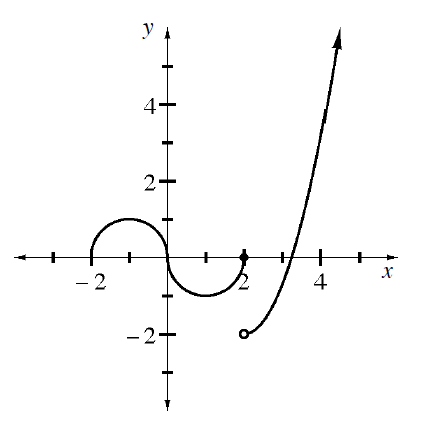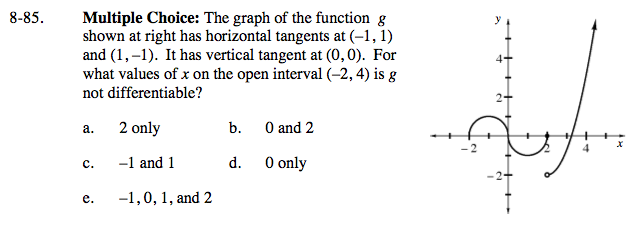### Home > CALC > Chapter 8 > Lesson 8.2.2 > Problem8-85

8-85.
1. Multiple Choice: The graph of the function g shown below has horizontal tangents at (−1, 1) and (1, −1). It has vertical tangent at (0, 0). For what values of x on the open interval (−2, 4) is g not differentiable? Homework Help ✎1. 2 only

2. 0 and 2

3. −1 and 1

4. 0 only

5. −1, 0, 1, and 2First of all, differentiability implies continuity. (That is to say a point of discontinuity has not derivative.) Secondly, the slope must agree from the left and the right.

Next, the value of the derivative must exist. (That is to say, the derivative must be finite.) Lastly, the value of the derivative must agree with the limit of the derivative at that point.